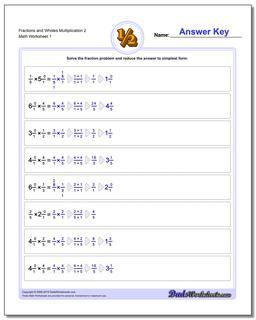# Math Worksheets: Fraction Multiplication: Fraction Multiplication: Fractions and Wholes Multiplication 2## Fractions and Wholes Multiplication 2

PropertyValue
DescriptionFractions and Wholes Multiplication 2: Basic multiplying fractions against wholes. Each problem on these worksheets has a single fraction multiplied by a whole value.
Resource TypeWorksheet